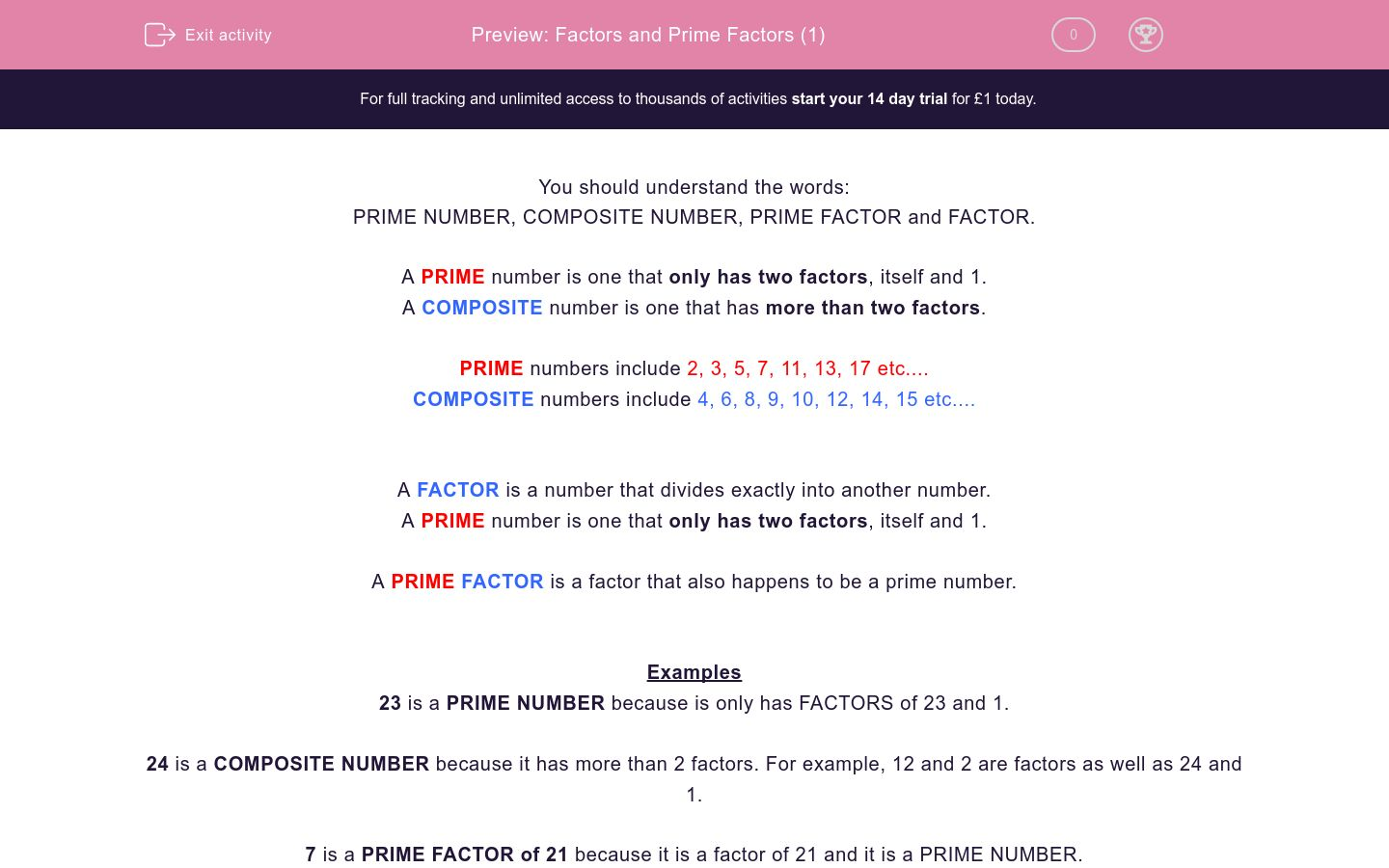# Factors and Prime Factors (1)

In this worksheet, students must complete the sentences using the correct vocabulary. Prime Numbers, Prime Factors and Composite Numbers should be understood.Key stage:  KS 2

Curriculum topic:   Maths and Numerical Reasoning

Curriculum subtopic:   Special Numbers

Difficulty level:### QUESTION 1 of 10

You should understand the words:

PRIME NUMBER, COMPOSITE NUMBER, PRIME FACTOR and FACTOR.

A PRIME number is one that only has two factors, itself and 1.

A COMPOSITE number is one that has more than two factors.

PRIME numbers include 2, 3, 5, 7, 11, 13, 17 etc....

COMPOSITE numbers include 4, 6, 8, 9, 10, 12, 14, 15 etc....

A FACTOR is a number that divides exactly into another number.

A PRIME number is one that only has two factors, itself and 1.

A PRIME FACTOR is a factor that also happens to be a prime number.

Examples

23 is a PRIME NUMBER because is only has FACTORS of 23 and 1.

24 is a COMPOSITE NUMBER because it has more than 2 factors. For example, 12 and 2 are factors as well as 24 and 1.

7 is a PRIME FACTOR of 21 because it is a factor of 21 and it is a PRIME NUMBER.

Complete the sentence.

23 is aprime number

composite number

prime factor

Complete the sentence.

24 is aprime number

composite number

prime factor

Complete the sentence.

3 is aof 9

prime number

composite number

prime factor

Complete the sentence.

5 is aof 10

prime number

composite number

prime factor

Complete the sentence.

21 is aprime number

composite number

prime factor

Complete the sentence.

29 is aprime number

composite number

prime factor

Complete the sentence.

27 is aprime number

composite number

prime factor

Complete the sentence.

11 is aof 22

prime number

composite number

prime factor

Complete the sentence.

6a prime factor of 30

is

is not

Complete the sentence.

5a prime factor of 30

is

is not

• Question 1

Complete the sentence.

23 is aprime number
EDDIE SAYS
23 is a prime number as it only has factors of 23 and 1.
• Question 2

Complete the sentence.

24 is acomposite number
EDDIE SAYS
24 is a composite number as it has more than 2 factors and is not prime.
For example, 8 and 3 are factors of 24.
• Question 3

Complete the sentence.

3 is aof 9

prime factor
EDDIE SAYS
3 is a factor of 9 and it is prime.
• Question 4

Complete the sentence.

5 is aof 10

prime factor
EDDIE SAYS
5 is a factor of 10 and it is prime.
• Question 5

Complete the sentence.

21 is acomposite number
EDDIE SAYS
21 is a composite number as it has more than 2 factors and is not prime.
For example, 7 and 3 are factors of 21.
• Question 6

Complete the sentence.

29 is aprime number
EDDIE SAYS
29 is a prime number as it only has factors of 29 and 1.
• Question 7

Complete the sentence.

27 is acomposite number
EDDIE SAYS
27 is a composite number as it has more than 2 factors and is not prime.
For example, 9 and 3 are factors of 27.
• Question 8

Complete the sentence.

11 is aof 22

prime factor
EDDIE SAYS
11 is a factor of 22 and it is prime.
• Question 9

Complete the sentence.

6a prime factor of 30

is not
EDDIE SAYS
6 is a factor of 30 but it is not a prime number.
• Question 10

Complete the sentence.

5a prime factor of 30

is
EDDIE SAYS
5 is a factor of 30 and it is a prime number.
---- OR ----

Sign up for a £1 trial so you can track and measure your child's progress on this activity.

### What is EdPlace?

We're your National Curriculum aligned online education content provider helping each child succeed in English, maths and science from year 1 to GCSE. With an EdPlace account you’ll be able to track and measure progress, helping each child achieve their best. We build confidence and attainment by personalising each child’s learning at a level that suits them.

Get started